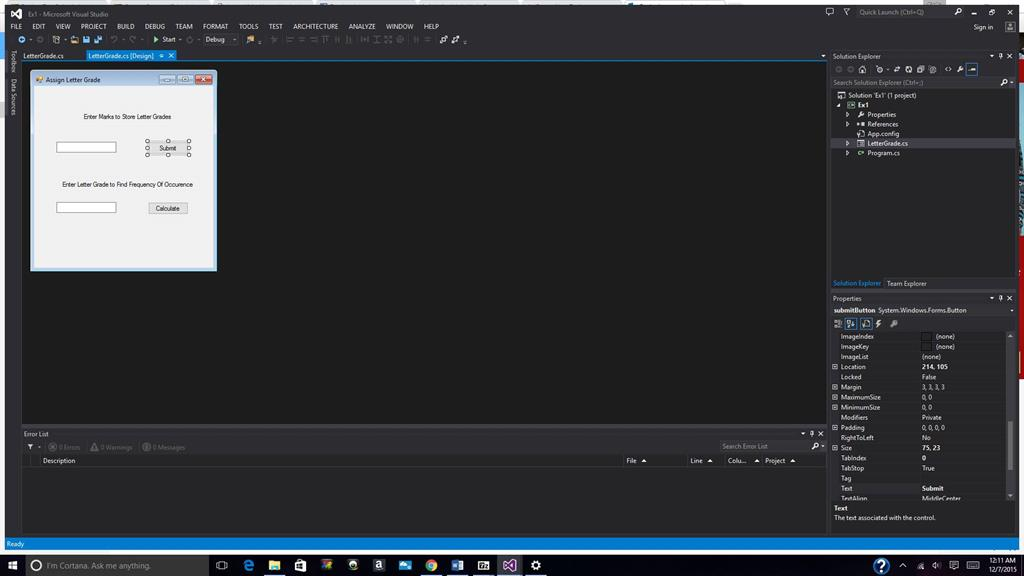Post

# Homework Question

Dec 7 2015 12:15 AMThis is the homework question....

For all elements in the List letterGrades a. If the element at index i is equal to grade, then increment noOfGrades by 1. This comparison should happen regardless of case, i.e., for p/P/f/F.This is what i have so far....

/*
* Calculate letter grades as P for Pass and F for Fail based on the marks obtained.
* Find the number of instances with the given grades.
*/
namespace Ex1
{
public partial class LetterGrade : Form
{
// Initialize string List
public List<string> letterGrades = new List<string>();
{
InitializeComponent();
}
private void submitButton_Click(object sender, EventArgs e)
{
double marks = double.Parse(marksTextbox.Text); //Declare variable marks and set
// If statements for marks
if (marks>0 && marks<=60)
{
}
else if (marks>60 && marks<=100)
{
}
else
{
MessageBox.Show("Not a valid marks");
}
marksTextbox.Clear();
}
private void calculateButton_Click(object sender, EventArgs e)
{
for (int i = 0; i < letterGrades.Count; i++)
{
if (grade == "P" || grade == "p") //Problem here, step 7a...could not initialize using [i]..tried using a workaround but it keeps total count..
{
}